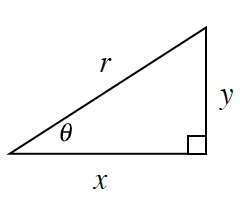### Home > INT2 > Chapter 9 > Lesson 9.3.1 > Problem9-91

9-91.Examine the diagram of the triangle at right.

1. Write an equation representing the relationship of the side lengths using only $x, y,$ and $r$.

Use the pythagorean theorem.

2. Write an expression for $\sin(θ)$. What is $\sin(θ)$ if $r = 1$?

If $r = 1$, then  $\sin(θ) = y$.

3. Write an expression for $\cos(θ)$. What is $\cos(θ)$ if $r = 1$?

If $r = 1$, then $\cos(θ) = x$.